# Colleges with the highest SAT scores in Arkansas

Top 10 colleges in Arkansas with the highest SAT scores
Looking for the colleges with the highest SAT scores in Arkansas? Well you're in luck! We've compiled a national college database and have created a list of the top 10 universities with the highest SAT scores in Arkansas. These are the schools whose applicants had the highest average SAT scores in Arkansas. And, since these tests are meant to determine academic prowess, they are arguably the schools with the most academically proficient students. You could even say these are the best colleges in Arkansas. We also include each college's ACT scores and acceptance rate so that you can see where you would have the easiest or hardest time getting in. Read on to find out more.

## Hendrix College SAT scores

The average SAT score for Hendrix College is 1260.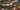The average SAT score of 1260 breaks down into:

• SAT math: 640

The average ACT score for Hendrix College is 27 and their acceptance rate is 79.8%.

## John Brown University SAT scores

The average SAT score for John Brown University is 1200.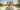The average SAT score of 1200 breaks down into:

• SAT math: 580

The average ACT score for John Brown University is 27 and their acceptance rate is 75.8%.

## University of Arkansas SAT scores

The average SAT score for University of Arkansas is 1195.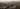The average SAT score of 1195 breaks down into:

• SAT math: 590

The average ACT score for University of Arkansas is 26 and their acceptance rate is 77.5%.

## Harding University SAT scores

The average SAT score for Harding University is 1180.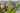The average SAT score of 1180 breaks down into:

• SAT math: 580

The average ACT score for Harding University is 26 and their acceptance rate is 67.9%.

## Ouachita Baptist University SAT scores

The average SAT score for Ouachita Baptist University is 1145.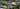The average SAT score of 1145 breaks down into:

• SAT math: 570

The average ACT score for Ouachita Baptist University is 25 and their acceptance rate is 63.6%.

## Lyon College SAT scores

The average SAT score for Lyon College is 1140.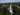The average SAT score of 1140 breaks down into:

• SAT math: 580

The average ACT score for Lyon College is 25 and their acceptance rate is 64.3%.

## University of Arkansas - Fort Smith SAT scores

The average SAT score for University of Arkansas - Fort Smith is 1110.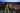The average SAT score of 1110 breaks down into:

• SAT math: 555

The average ACT score for University of Arkansas - Fort Smith is 22 and their acceptance rate is 56.3%.

## Southern Arkansas University Magnolia SAT scores

The average SAT score for Southern Arkansas University Magnolia is 1103.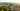The average SAT score of 1103 breaks down into:

• SAT math: 540

The average ACT score for Southern Arkansas University Magnolia is 22 and their acceptance rate is 68.8%.

## Arkansas Tech University SAT scores

The average SAT score for Arkansas Tech University is 1100.The average SAT score of 1100 breaks down into:

• SAT math: 560

The average ACT score for Arkansas Tech University is 22 and their acceptance rate is 90.2%.

## University of the Ozarks SAT scores

The average SAT score for University of the Ozarks is 1070.The average SAT score of 1070 breaks down into:

• SAT math: 530